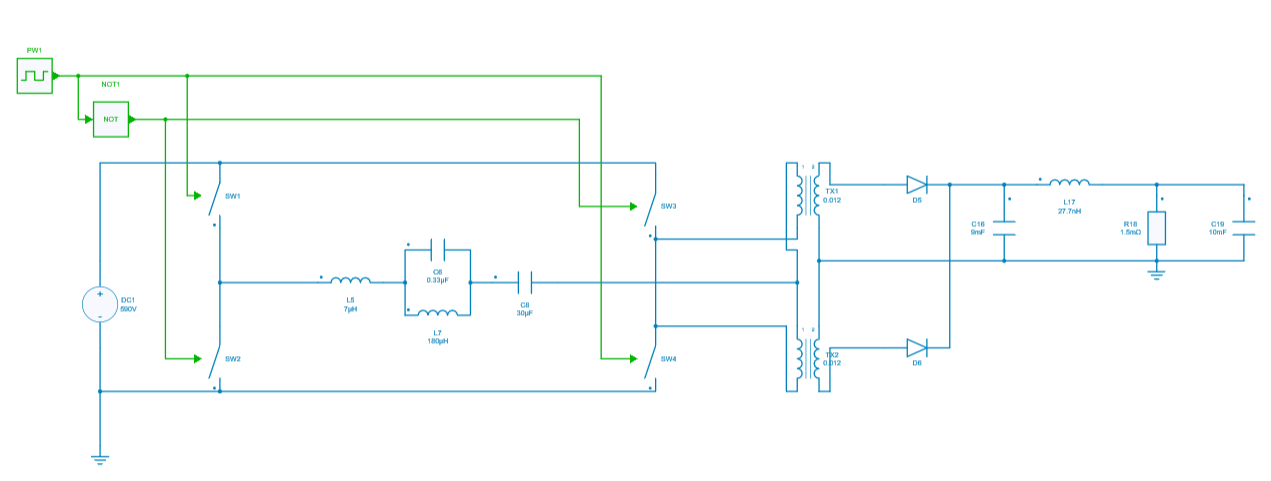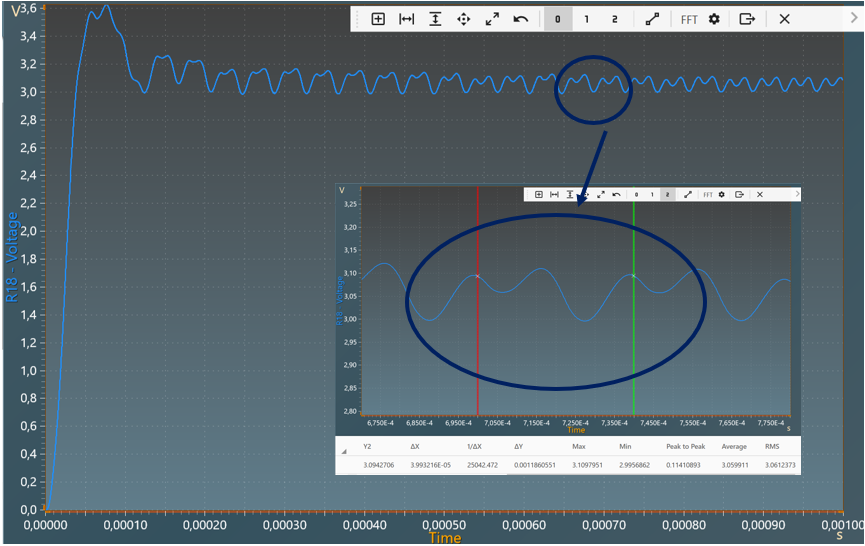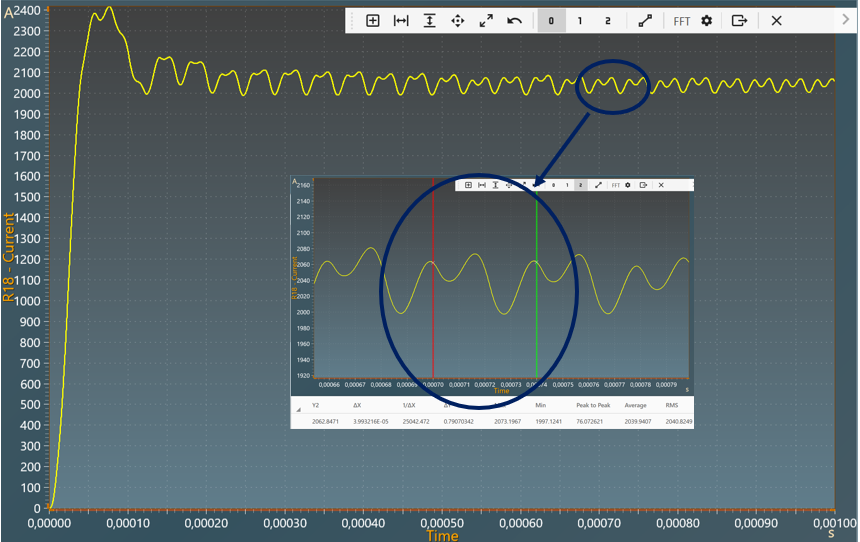Resources

# DC-DC Resonant converter

This example shows a DC-DC Resonant Converter with:

• an input voltage of 590 V,
• an output voltage of 3.06 V,
• a power of 6.242 kW transferred at the output resistor.

The Resonant converter is a power electronic DC-DC converter which use a resonant circuit with a combination of capacitors and inductors in order to resonate at a particular frquency. This type of converter reduces the switching losses & switching noise with the help of soft switching and is mainly used in high-power applications such as telecoms, servers and high power consumer electronics. A resonant converter consists of 3 parts:

1. a switch network (DC-AC converter),
2. a resonant tank/resonant circuit (Inductor and capacitors);
3. and a rectifier network (AC/DC converter).

Finally, resonant converter provides high efficiency at high power also the overall size of the converter decreases.

## Model

In this model, all components are ideal.The inverter network provides AC signal which behave as square wave having sharp edges with multiples harmonics.

Then the resonant converter basically filters out all the harmonics present in the signal and tries to deliver the 1st harmonic at the output.

In that sense, the current flowing through this tank circuit will be smooth out, feed into the transformer (galvanic isolation) and AC signal will be filtered from the rectifier.

## Switch control

Inverter network:

The switch model can be directly connected with a control signal. For this control, we use a PWM block with an amplitude of 1, a duty cycle of 0.5 and a frequency of 25KHz.

Here are the values of the other components :

Resonant tank:

• inductor L5 : 7 µH and initial current = 0A
• capacitor C6: 0.33 µF and initial voltage = 0V
• inductor L7: 180 µH and initial current = 0A
• capacitor C8: 30 µF and invitial voltage = 0V

• capacitor C16: 9 mF and initial voltage = 0V
• capacitor C19: 10 mF and initial voltage = 0V
• inductor L17: 27.7 nH and initial current = 0A
• resistor R18: 1.5 $$\Omega$$
• diode D5: Rd = 0 $$\Omega$$ and Forward voltage = 0V
• diode D6: Rd = 0 $$\Omega$$ and Forward voltage = 0V
• transformer TX1: ratio $$\frac{V2}{V1} = 0.012$$
• transformer TX2: ratio $$\frac{V2}{V1} = 0.012$$

## Simulation

The results below show the output voltage across R18 with a duty cycle= 0.5. A zoom shows a measure of the average value of this voltage : 3.06 V.The results below show the output current through R18 with a duty cycle= 0.5. A zoom shows a measure of the average value of this current : 2040 A.